﻿ Result Correlation of Experimental and Numerical Modal Analysis of a Steel Beam

### Result Correlation of Experimental and Numerical Modal Analysis of a Steel Beam

Peter Pavelka, Róbert Huňady, Martin Hagara

American Journal of Mechanical Engineering

## Result Correlation of Experimental and Numerical Modal Analysis of a Steel Beam

Peter Pavelka1,, Róbert Huňady1, Martin Hagara1

1Department of Applied Mechanics and Mechanical Engineering, Faculty of Mechanical Engineering, Letná 9, 042 00 Košice, Slovakia

### Abstract

The paper deals with an experimental and a numerical investigation of dynamic properties of the structure. Object of interest is a steel rod with circle section. The modal parameters, such as mode shapes and natural frequencies, are obtained by two different ways: experimentally by using experimental modal analysis and numerically by finite element analysis which were computed in NX Nastran solver. The results from both methods are compared in NX through the correlation function. A relation between measured mode shapes and computed mode shapes is expressed by Modal Assurance Criterion. This process of comparing is appropriate for verification of experimental approaches.

• Peter Pavelka, Róbert Huňady, Martin Hagara. Result Correlation of Experimental and Numerical Modal Analysis of a Steel Beam. American Journal of Mechanical Engineering. Vol. 4, No. 7, 2016, pp 376-379. http://pubs.sciepub.com/ajme/4/7/26
• Pavelka, Peter, Róbert Huňady, and Martin Hagara. "Result Correlation of Experimental and Numerical Modal Analysis of a Steel Beam." American Journal of Mechanical Engineering 4.7 (2016): 376-379.
• Pavelka, P. , Huňady, R. , & Hagara, M. (2016). Result Correlation of Experimental and Numerical Modal Analysis of a Steel Beam. American Journal of Mechanical Engineering, 4(7), 376-379.
• Pavelka, Peter, Róbert Huňady, and Martin Hagara. "Result Correlation of Experimental and Numerical Modal Analysis of a Steel Beam." American Journal of Mechanical Engineering 4, no. 7 (2016): 376-379.

 Import into BibTeX Import into EndNote Import into RefMan Import into RefWorks

### 1. Introduction

The modal tests are being performed for several reasons. One of them is a process of comparison experimentally obtained data from measurement with data from finite element analysis or with other theoretical methods. Investigation of damping value can be the next reason. It is useful to refine computational model. For example, if it is assumed the vicious damping (Rayleigh damping), when damping is a linear combination of mass and stiffness.

Other reason can be to find out causes, why the construction vibrates. Answering the question of whether the vibration is caused by resonance and what the excited modal shape looks like provide modal analysis.

1.1. Experimental Modal Analysis

Experimental modal analysis is a part of mechanics and it used for knowledge of the dynamic behavior of vibrating structures. An aim of this process is to determine modal parameters such as natural frequencies, mass, stiffness, damping and mode shapes for a linear and time-invariant system . Foundation of experimental modal analysis is measuring response and excitation force. Ratio of response to excitation provides frequency response function (FRF). By analysis of the measured FRFs are determined modes of vibration. Every each mode is defined by natural frequency, mode shape and damping. In general FRF can be expressed by: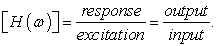(1)

For a single element of FRF matrix can be described by equation: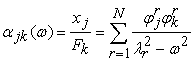(2)

where: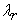is eigenvalue of the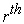mode (natural frequency + modal damping),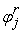is the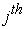element of thenatural shapes vector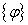,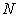is number of modes.

1.2. Numerical Modal Analysis

Analytical solution solves an eigenvalue problem by solving equations. The results of numerical modal analysis are to get the natural frequency and their relevant mode shape. The equation of motion for an N degrees of freedom structure is given by: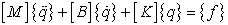(3)

where: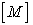is mass matrix,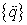is vector of acceleration,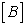is damping matrix,is vector of velocity,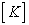is stiffness matrix,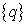is vector of displacement and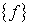is vector of excitation force.

### 2. Determination the Modal Parameters by Experimental Modal Analysis

The aim of the experiment was to establish modal parameters of a steel rod with circular cross section (diameter 22 mm, length 800 mm). The structure was a freely suspended. The nineteen measurement points were defined and marked on the rod (Figure 1). The eighteen of them represented input degrees of freedom in which the structure was excited by impact hammer (Bruel & Kjaer, type 8206 with plastic tip). These points were equally spaced apart. The last one (point 19) was the reference output DOF (Figure 2) in which the responses were measured by accelerometer (Bruel & Kjaer, type 4507 B 004). This point was located between the points 17 and 18.Download asVeiw figureFigures index
Figure 1. Steel rod with marked DOFs

A geometrical model and a model with all degrees of freedom are shown in Figure 2. There can be seen two different markers, where black-green hammers indicate the places and directions in which the structure was excited and red arrow indicates the position and orientation of the accelerometer. Experimental modal analysis was implemented by measuring system PULSETM.Download asVeiw figureFigures index
Figure 2. Geometrical model of the structure

The frequency measuring range was set from 0 to 10 kHz with 3 linear averaging of FRFs. The frequency range was split by 6400 discrete lines.Download asVeiw figureFigures index
Figure 3. Measuring equipment

The FRF matrix was created from the set of the measured data. Measurement was evaluated in software Pulse Reflex®. Complex mode identificator function was calculated by singular value decomposition of FRF matrix. Its magnitude spectrum is shown in Figure 3. There are twelve peaks which can be easily identified. Each of them indicates presence mode of vibration, i.e. the resonance frequency of the rod.

Rational fraction polynomial method was used to estimate of modal parameters in so-called curve fitting process. Natural frequencies for each mode are listed in Table 1. Damping values are listed only for interest. Their relevant mode shapes are shown in Figure 5 – Figure 16 on the left side.Download asVeiw figureFigures index
Figure 4. Complex mode identificator function from measurement

### 3. Numerical Modal Analysis

The numerical simulation was performed in Siemens NX 10.0. Geometrical model was represented by one line with length of 800 millimeter. Beam elements (CBEAM) were used to create finite element mesh. The circular cross section with radius of 11 mm and material properties of the steel were assigned to all elements. The number of elements was 181. Solver NX Nastran (Solution type: SOL 103 Real Eigenvalues) was used for the solution. Boundary conditions were not defined what considered to the analysis of free oscillation.

The results of the simulation are natural frequencies and mode shapes. The natural frequencies are listed in Table 2; the mode shapes are shown in Figure 5 – Figure 16 on the right side.

### 4. Comparison of Results of Numerical and Experimental Modal Analysis

The measured frequencies and the computed frequencies are in good agreement. It can be seen in Table 3 that the first three measured frequencies are higher than computed. It is caused by the stiffness of support used in the experiment. Other frequencies are lower due to higher stiffness of FE model.

#### Table 3. Shift of the natural frequencies

On a base of visual assessment it can be said that the measured mode shapes are also in good agreement with the computed shapes (Figure 5 – Figure 16).Download asVeiw figureFigures index
Figure 5. The 1st mode shape (measured on the left side, computed on the right side)Download asVeiw figureFigures index
Figure 6. The 2nd mode shape (measured on the left side, computed on the right side)Download asVeiw figureFigures index
Figure 7. The 3rd mode shape (measured on the left side, computed on the right side)Download asVeiw figureFigures index
Figure 8. The 4th mode shape (measured on the left side, computed on the right side)Download asVeiw figureFigures index
Figure 9. The 5th mode shape (measured on the left side, computed on the right side)Download asVeiw figureFigures index
Figure 10. The 6th mode shape (measured on the left side, computed on the right side)Download asVeiw figureFigures index
Figure 11. The 7th mode shape (measured on the left side, computed on the right side)Download asVeiw figureFigures index
Figure 12. The 8th mode shape (measured on the left side, computed on the right side)Download asVeiw figureFigures index
Figure 13. The 9th mode shape (measured on the left side, computed on the right side)Download asVeiw figureFigures index
Figure 14. The 10th mode shape (measured on the left side, computed on the right side)Download asVeiw figureFigures index
Figure 15. The 11th mode shape (measured on the left side, computed on the right side)Download asVeiw figureFigures index
Figure 16. The 12th mode shape (measured on the left side, computed on the right side)

Modal Assurance Criterion (MAC) was used for quantitative assessment of the results. MAC indicate compliance rate between sets of data. For example, if it is needed to compare experimentally measured mode shape and theoretically predicted mode shape, as in this case. The MAC value is computed by equation: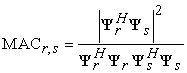(4)

where: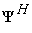is complex conjugate transpose (Hermitian) of the given modal vector,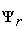is modal vector of mode r,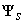is modal vector of mode s .

MAC value is a scalar parameter between zero and one. The value near one indicates high level of compliance between corellated mode shapes. Vice versa, the value near zero says about different mode shapes.

MAC indicator shows high level of compliance. With increasing frequency decreases MAC value (Table 4). This is because mode shape is more difficult with increasing frequency. The shape, which is measured experimentally, is dependent on density of defined degrees of freedom. For more detailed description of shape, it is needed to use more measuring points. In ideal case, we should use infinitely many of measuring points.

### 5. Conclusion

This paper deals with experimental modal analysis of steel rod with circle section. The modal parameters are estimated for free supported structure. Subsequently, numerical simulation was carried out by using finite element method. The results obtained by both ways were compared. The comparison of natural frequencies showed the relatively small differences that are acceptable with respect to differences in boundary conditions in the experiment and the numerical simulations. The support used during the measurement had impact to dynamic behavior of the structure. It is obvious that a free support is difficult to achieve in the real space. The results also showed the good match between measured and computed mode shapes.

### Acknowledgements

This work was supported by project VEGA1/0393/14 and VEGA 1/0751/16.

### References

  Trebuňa, F., Šimčák, F., Huňady, R. Vibration and modal analysis of mechanical systems, (In Slovak), Typopress, Košice, 2012.In article  Ewins D. J. Modal testing – Theory, practice and application. 2. edition, Wiley, (2000).In article  Bilošová. A. Experimental modal analysis, (In Czech), VŠB TU Ostrava, 2011.In article  Siemens Documentation of softvare NX 10.0.In article  Pavelka, P., Huňady, R., Hagara, M., Trebuňa, F. “Reciprocity in Experimental Modal Analysis”, American Journal of Mechanical Engineering Vol. 3, pp 252-256, No. 6, 2015.In article